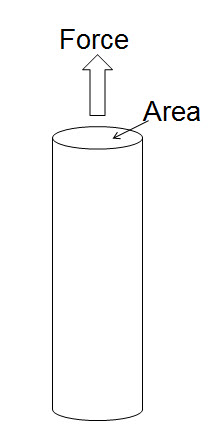## Basic of Drillpipe Tensile Capacity and Its Calculation

This article demonstrates basic knowledge understanding of tensile capacity of the drill pipe and some calculation. First of all we need to know basic of material strength and for our case is strength of metal.There are few simple terminologies which we would like to explain.

## Stress (σ)

Stress (σ) equals to force divided by cross sectional area of the material (F/A). For our case, we will discuss about only stress in tensile because the drill pipe is almost always designed to work in a tensile condition.

### Stress (σ) = F/AFigure 1 – Stress (σ) = F/A